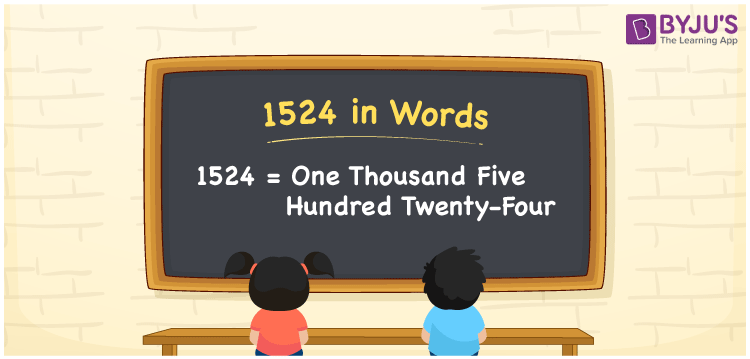# 1524 in Words

The number 1524 in words is One Thousand Five Hundred Twenty-Four. For instance, the cost of a designer gown is Rs. 1524, then you can say, “The cost of a designer gown is Rupees One Thousand Five Hundred Twenty-Four”. 1524 is a cardinal number as it indicates the specific amount. Let us learn in detail the conversion of the number 1524 into words and its interesting facts in this article.

 1524 in Words One Thousand Five Hundred Twenty-Four One Thousand Five Hundred Twenty-Four in numerical form 1524

## 1524 in English Words

In Maths, we write numbers in words using the letters of the English alphabet. Therefore, we can read the number 1524 in English as One Thousand Five Hundred Twenty-Four.## How to Write 1524 in Words?

Let us learn how to convert the number 1524 in word form using a place value table. The below table represents the place value chart for the number 1524.

 Thousands Hundreds Tens Ones 1 5 2 4

Hence, we can write the expanded form as:

1 x Thousand + 5 x Hundred + 2 x Ten + 4 x One

= 1 x 1000 + 5 x 100 + 2 x 10 + 4 x 1

= 1000 + 500 + 20 + 4

= 1524

= One Thousand Five Hundred Twenty-Four

Therefore, 1524 in words is written as One Thousand Five Hundred Twenty-Four

Interesting way of writing 1524 in words

1 = One

15 = Fifteen

152 = One Hundred and Fifty-Two

1524 = One Thousand Five Hundred Twenty-Four

Thus, the word form of the number 1524 is One Thousand Five Hundred Twenty-Four

1524 is a natural number that is the successor of 1523 and the predecessor of 1525

• 1524 in words – One Thousand Five Hundred Twenty-Four
• Is 1524 an odd number? – No
• Is 1524 an even number? – Yes
• Is 1524 a perfect square number? – No
• Is 1524 a perfect cube number? – No
• Is 1524 a prime number? – No
• Is 1524 a composite number? – Yes

## Frequently Asked Questions on 1524 in Words

Q1

### How to write 1524 in English words?

1524 in English words is written as One Thousand Five Hundred Twenty-Four.
Q2

### Simplify 1000 + 524, and express in words.

Simplifying 1000 + 524, we get 1524. Therefore, the number 1524 in words is One Thousand Five Hundred Twenty-Four.
Q3

### 1524 is a composite number. True or False.

True, the number 1524 is a composite number.